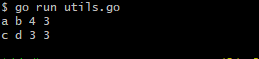• 取整运算又分为向上取整向下取整， 函数分别为math.Ceil(x)和math.Floor(x) 2.1可以对数x分别向上取整向下取整。 2.2可以对数x+0.5，然后向下取整 2.3可以对数x-0.5，然后向上取整 值得注意的是取整后返回的数值...
1.Golang中没有float64转int的调用函数，但是给出了取整运算的函数。
2.浮点型转int类型，本质是取整运算。假设x为float64类型
取整运算又分为向上取整和向下取整，
函数分别为math.Ceil(x)和math.Floor(x)
2.1可以对数x分别向上取整和向下取整。
2.2可以对数x+0.5，然后向下取整
2.3可以对数x-0.5，然后向上取整
值得注意的是取整后返回的数值依然是float64类型，需要用int类型强制转换!
实例
package main
import (
“fmt”
“math”
)
func main(){
x:= 3.1415
//分别取整
a:=int(math.Ceil(x))
b:=int(math.Floor(x))
fmt.Println(“a”,“b”,a,b)
//四舍五入
c:=int(math.Ceil(x-0.5))
d:=int(math.Floor(x+0.5))
fmt.Println(“c”,“d”,c,d)
}展开全文golang
• 废话少叙，直接上code： 首先，不得不提醒大家一个容易被忽视或者搞混的问题——一般的，0.5这种末尾是5的小数...向上取整：math.ceil() import math math.ceil(-0.5) &gt;&gt;&gt; 0 math.ceil(-...
废话少叙，直接上code：

首先，不得不提醒大家一个容易被忽视或者搞混的问题——一般的，0.5这种末尾是5的小数，四舍五入取整应进位。这个进位的意思是：-0.5 → -1；0.5 → 1.即正负情况不同，都向着远离0，使得绝对值更大的方向进位

向上取整：math.ceil()

import math

math.ceil(-0.5)
>>> 0

math.ceil(-0.9)
>>> 0

math.ceil(0.3)
>>> 1

如code所见，math.ceil()严格遵循向上取整，所有小数都是向着数值更大的方向取整，不论正负数都如此

四舍五入：round()

round(-2.5)
>>> -2

round(-1.5)
>>> -2

round(-0.5)
>>> 0

round(0.5)
>>> 0

round(1.5)
>>> 2

round(2.5)
>>> 2

如code所示，round()当不传入第二个参数时默认取整，具体就是按照四舍五入来。但值得一提的是这里对小数末尾为5的处理方法：当末尾的5的前一位为奇数：向绝对值更大的方向取整（比如-1.5、1.5处理结果）；当末尾的5的前一位为偶数：去尾取整（比如-2.5，-0.5，0.5和2.5的处理结果）。

向下取整：math.floor()

math.floor(-0.3)
>>> -1

math.floor(0.9)
>>> 0

简单且忠实地向下取整，不再讨论

两个有趣且特殊的Python取整：int()、整除"//"

int()

int(-0.5)
>>> 0

int(-0.9)
>>> 0

int(0.5)
>>> 0

int(0.9)
>>> 0

一句话总结：int()函数是“向0取整”，取整方向总是让结果比小数的绝对值更小

"//"

(-1) // 2  # -0.5
>>> -1

(-3) // 2  # -1.5
>>> -2

1 // 2    # 0.5
>>> 0

3 // 2    # 1.5
>>> 1

一句话总结：“整除”符号运算将结果忠实地向下取整，与math.floor()处理结果一样

总结一下：

向上取整：math.ceil()
向下取整：math.floor()、整除"//"
四舍五入：round()——奇数向远离0取整，偶数去尾取整；或言之：奇数进位，偶数去尾
向0取整：int()
由于最近在做算法题，许多算法题都要涉及(0-1)/2这类的边界计算，这时候我们想让这个-0.5取整至0，而且想让(4-1)/2的结果1.5取整至1，即正数时向下取整，负数时向上取整，总而言之就是向0取整，这时候我们可以用int()

展开全文• 值得注意的是，题目里有一个要求是取给定人数×150%（向下取整）的分数作为面试分数线，这里顺便提一下c++里面的两个取整函数：地板函数（floor）和天花板函数（ceil）。 举个栗子 floor(10.5) == 10 floor(-10.5) =...
原题点这里
这道题主要考察的是排序，有个方便的方法就是定义一个结构体，存储每个志愿者的报名号和笔试分数，然后定义一个排序就OK了
值得注意的是，题目里有一个要求是取给定人数×150%（向下取整）的分数作为面试分数线，这里顺便提一下c++里面的两个取整函数：地板函数（floor）和天花板函数（ceil）。
举个栗子
floor(10.5) == 10    floor(-10.5) == -11
ceil(10.5) == 11    ceil(-10.5) ==-10

使用floor函数。floor(x)返回的是小于或等于x的最大整数。
使用ceil函数。ceil(x)返回的是大于x的最小整数。
不得不说点：/ % 四舍五入 向上取整（ceil()） 向下取整（floor）
然后是这道题的代码
#include <bits/stdc++.h>
using namespace std;
struct volunteer{
int number;
int score;
}a;
bool cmp(volunteer a,volunteer b)
{
if(a.score>b.score) return true;
else if(a.score==b.score) return a.number<b.number;
else return false;
}

int main(){
int n;
double m;
cin>>n>>m;
for(int i=0;i<n;i++){
cin>>a[i].number>>a[i].score;
}
sort(a,a+n,cmp);
int r=floor(m*1.5)-1;
int lower=a[r].score;
int j;
for(j=0;a[j].score>=lower;j++);
cout<<lower<<" "<<j<<endl;
for(int i=0;i<j;i++)
cout<<a[i].number<<" "<<a[i].score<<endl;
return 0;
}



展开全文结构体 排序
• = 4.0 至于int()的整数截断具体原理，我不是很了解。 依据见下面的代码： for i in range(20): numstr = '3.' + '9' * i print(f"float('{numstr}') = {float(numstr)}") if float(numstr) == 4.0: break 运行结果：...
我认为是python浮点数精度限制（浮点数的位数是有限的）导致的，3.9999999999999999999实际上与浮点数4.0从计算机表示角度是同一个数。实际上：float('3.9999999999999995') = 3.9999999999999996float('3.9999999999999996') = 3.9999999999999996float('3.9999999999999997') = 3.9999999999999996float('3.9999999999999998') = 4.0float('3.9999999999999999') = 4.0至于int()的整数截断具体原理，我不是很了解。依据见下面的代码：for i in range(20):numstr = '3.' + '9' * iprint(f"float('{numstr}') = {float(numstr)}")if float(numstr) == 4.0:break运行结果：float('3.') = 3.0float('3.9') = 3.9float('3.99') = 3.99float('3.999') = 3.999float('3.9999') = 3.9999float('3.99999') = 3.99999float('3.999999') = 3.999999float('3.9999999') = 3.9999999float('3.99999999') = 3.99999999float('3.999999999') = 3.999999999float('3.9999999999') = 3.9999999999float('3.99999999999') = 3.99999999999float('3.999999999999') = 3.999999999999float('3.9999999999999') = 3.9999999999999float('3.99999999999999') = 3.99999999999999float('3.999999999999999') = 3.999999999999999float('3.9999999999999999') = 4.0
展开全文• 向上取整1.1 函数解析1.2 举例 首先我们需要知道C标准库中是有向上/取整的函数的，需要引入math.h，但是是否有四舍五入的函数（即round()函数），取决于你的编译器是否支持C99标准！不过不用担心，即使你的编译器...
• C语言有以下几种取整方法：1、直接赋值给整数变量。如：int i = 2.5; 或 i = (int) 2.5;这种方法采用的是舍去小数部分。2、C/C++中的整数除法运算符"/"本身就有取整功能(int / int)...3、使用floor函数，向下取整。f...
• 这个记起来比较好记，ceil是天花板的意思，floor是地板的意思，通过这个我们就能很容易的区别哪个是向上取整，哪个是向下取整 示例如下： public class Test { public static void main(String[] args) { ...
• floor()函数：向下取整，取小于等于这个数的最大整数 ceil()函数：向上取整，取大于等于这个数的最小整数 import Foundation extension Double { func intValue() -&amp;amp;gt; Int { return Int(self...floor ceil
• Java中向上取整向下取整 在进行一些数据处理的时候，经常需要对结果进行向上或向下的取整操作，Java有专门的方法支持： 向上取整用Math.ceil(double a) 向下取整用Math.floor(double a) 例如： double a=30; ...
• //向下取整 float downF1 = 1.2; float downF2 = 1.6; int downI1, downI2; downI1 = floorf(downF1); downI2 = floorf(downF2); //向上取整 ...
• ## 除法的向上取整和向下取整

万次阅读 多人点赞 2019-06-08 11:29:32
向上取整：比自己大的最小整数。 两个数相除向上取整：(a + b - 1) / b。比如：(13 / 3) = 4。向上取整：(13 + 3 - 1) / 3 = 5。另外还可以用C++中的ceil()函数：ceil (13 / 3) = 5. 向下取整：比自己小的最大整数。...
• 在PHP中，一般来说如果需要向上取整向下取整。用 ceil()、floor() 函数就可以。但是如果对金额的向上、向下取整要怎么处理那（金额一般都是要保留两位小数的）。 向上取整 /** * 默认保留2位(金额向上取整算法) ...php
• 向上取整向下取整四舍五入 向上取整 判断a是否等于（int）a，如果不相等，说明a是一个＞（int）a的实数，所以将（int）a + 1就能得到a向上取整的结果。 向下取整 强制转换为int型，就可以达到向下取整的效果，如： ...
• // 向下取整 scala.math.floor(7.5) 结果： Double = 7.0 // 向上取整 scala.math.floor(7.5) 结果： Double = 8.0 // 四舍五入 scala.math.round(7.5) 结果： Long = 8 round 将long和double转化为long型 将float...
• JAVA Math函数向上取整向下取整,向上取整用Math.ceil(double a)向下取整用Math.floor(double a)举例：double a=35; double b=20; double c = a/b; System.out.println(“c===>”+c); //1.75 System.out....java 函数 math
• 向上取整 不管四舍五入的规则 只要后面有小数前面的整数就加1向下取整 不管四舍五入的规则 只要后面有小数忽略小数 给定 4.9调用用向下取整函数 得到的是 4调用用向上取整函数 得到的是 5 Word->插入->符号...
• python 向上取整ceil 向下取整floor 四舍五入round，相面给出源码示例. import math #向上取整 print "math.ceil---" print "math.ceil(2.3) => ", math.ceil(2.3) print "math.ceil(2.6) => ", math.ceil(2.6) #...python math 四舍五入
• 向上取整：math.ceil() 向下取整：math.floor()、整除"//" 四舍五入：round() 向0取整：int() 注：round()——针对小数点后.5的情况：奇数向远离0取整，偶数去尾取整；或言之：奇数进位，偶数去尾。之所以出现...python
• 向上取整：比自己大的最小整数。 向下取整：比自己小的最大整数。
• 文章目录四舍五入向上取整向下取整 四舍五入 a = 3.5 print(round(a)) 向上取整 import math a = 3.1 print(math.ceil(a)) 向下取整 a = 3.4 print(int(a))python
• 向下取整。floor(x)返回的是x的整数部分。如： floor(2.5) = 2 floor(-2.5) = -3 ceil函数 向上取整。ceil(x)返回的是不大于x的最小整数。如： ceil(2.5) = 2 ceil(-2.5) = -2 floor和ceil对于正数没有区别，但是...
• 关于向上取整向下取整知识整理 向下取整函数f(x)=⌊x⌋f(x)=\lfloor x\rfloorf(x)=⌊x⌋ 是单调递增的 ，向上取整函数f(x)=⌈x⌉f(x)=\lceil x\rceilf(x)=⌈x⌉也是单调递增的。 对任意整数n， ⌈n2⌉+⌊n2⌋=n\...
• 向下取整：Math.floor(); System.out.println(5/2);//2 结果是整型 System.out.println(5.0/2.0);//2.5 结果是浮点数 System.out.println((int)Math.ceil(5/2));//2 5/2结果是整型2，向上取整是2 ...1024程序员节
• //向上取整 floor(m) //向下取整 实现： 向下取整：a / b 向上取整：(a + (b - 1)) / b 四舍五入：int(a×1.0 / b + 0.5) 之所以在向上取整时，分子部分要减去1，是为了避免出现，a 能被 b 整......